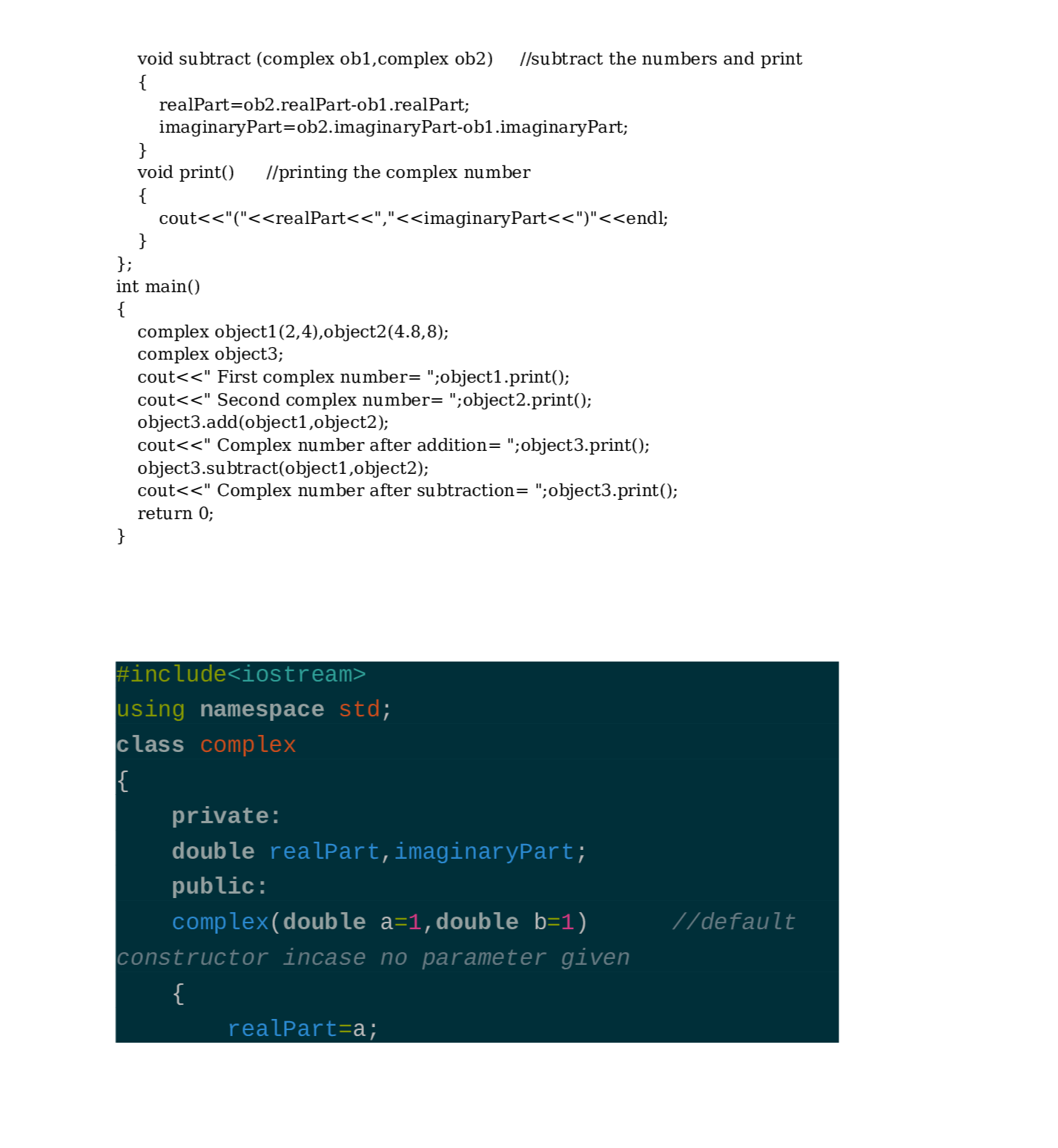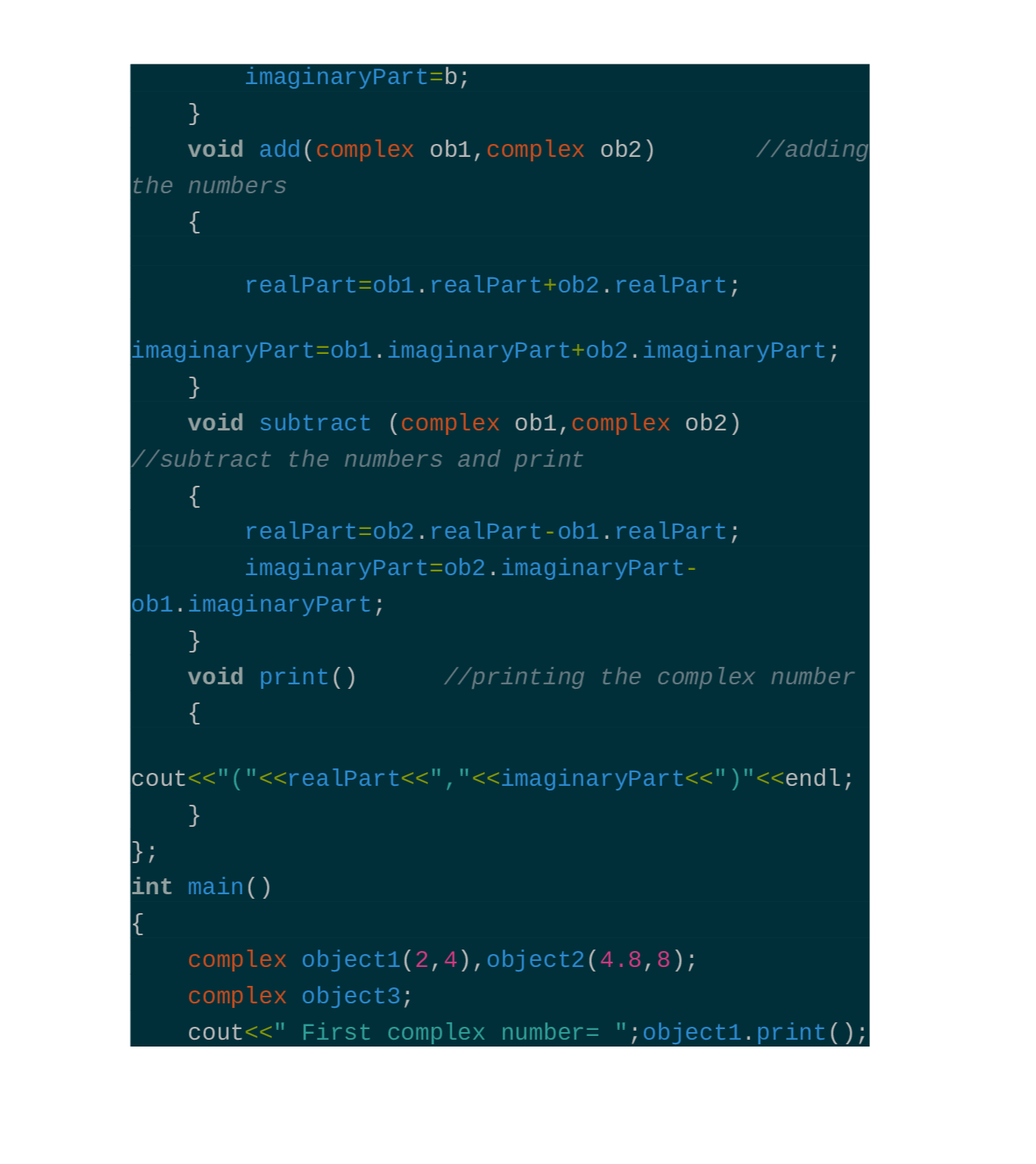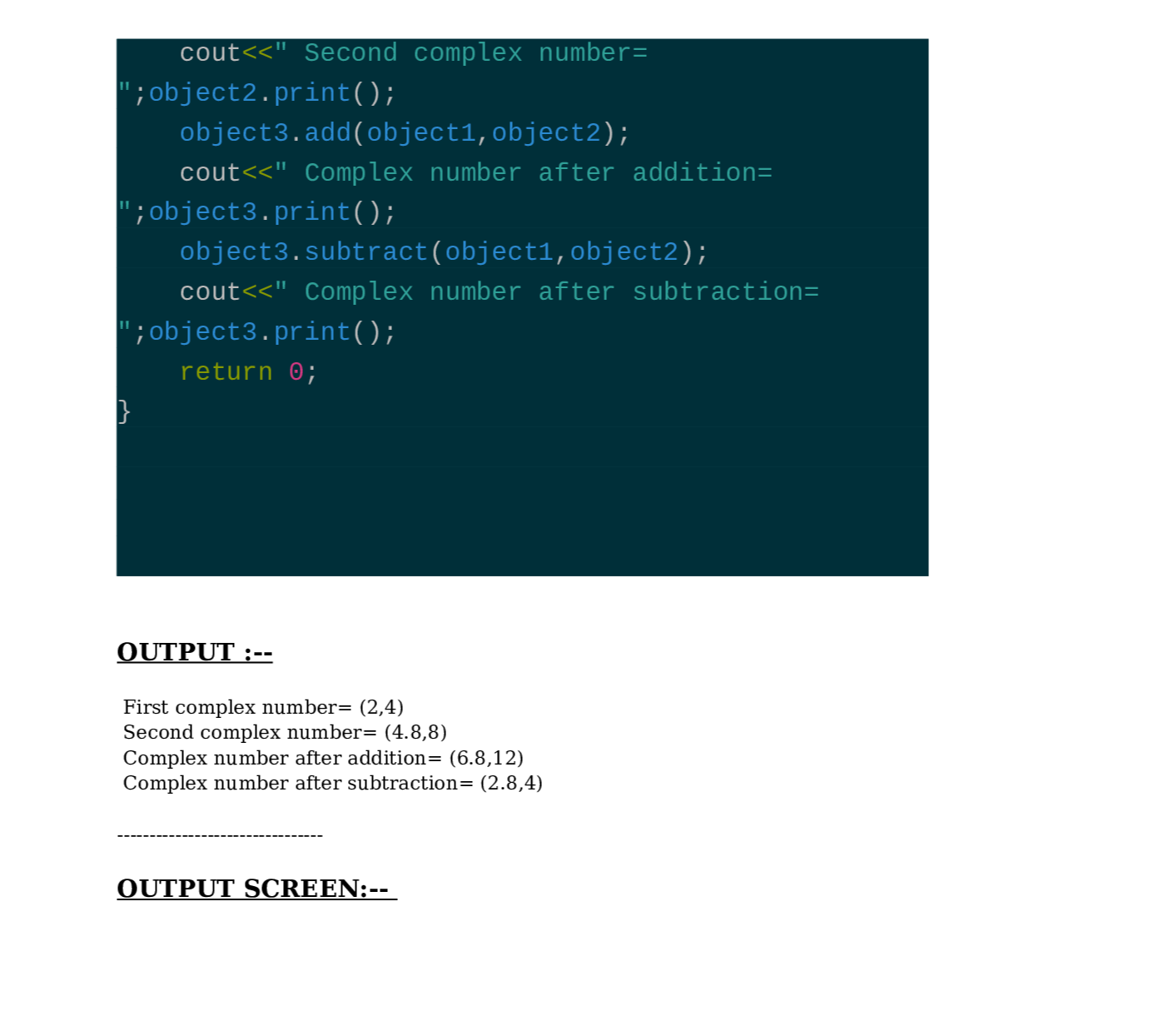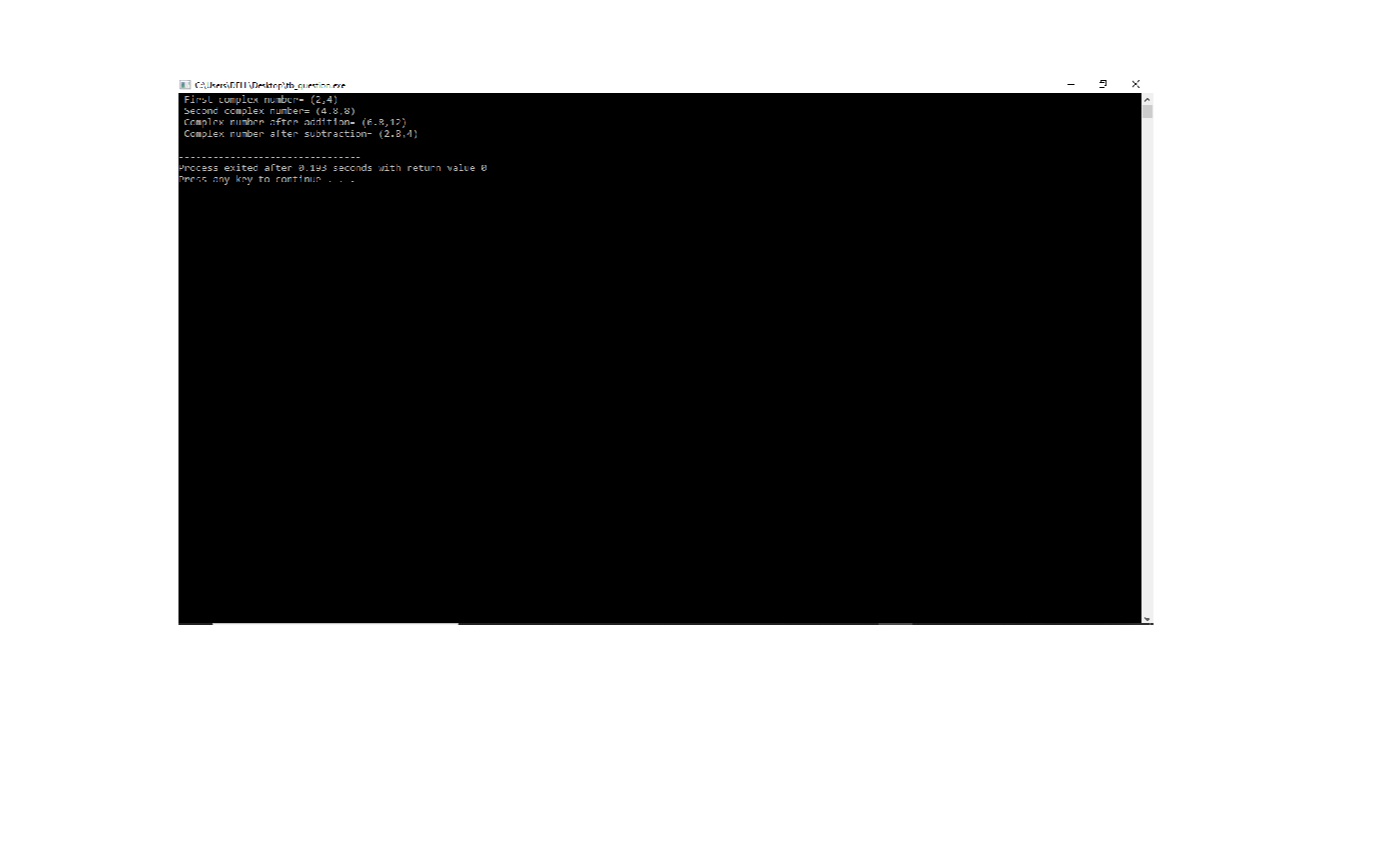Question

C/C++

2) Create a class called Complex for performing arithmetic with complex numbers.

Write a program to test your class. Complex numbers have the form

realPart + imaginaryPart * i

Use double variables to represent the private data of the class. Provide a constructor that enables an object of this class to constructor should contain default values in case no initializers are provided. Provide public member functions that perform the following tasks:initialized when it's declared. The

1. a) Adding two Complex numbers: The real parts are added together and the imaginary parts are added together.

2. b) Subtracting two Complex numbers: The real part of the right operand is subtracted from the real part of the left operand, and the imaginary part of the right operand is subtracted from the imaginary part of the left operand.

3. c) Printing Complex numbers in the form (a, b), where a is the real part and b is the imaginary part.Verified### Submit query